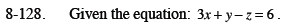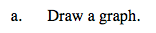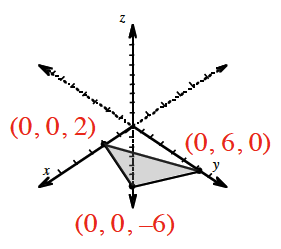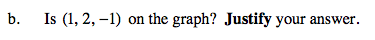### Home > CCA2 > Chapter 8 > Lesson 8.3.1 > Problem8-128

8-128.
1. Given the equation: 3x + yz = 6. Homework Help ✎

1. Draw a graph.Notice that there are three variables. Find the three intercepts.Does the point make the equation true?

Yes, it is a solution to the equation.